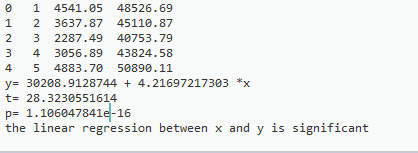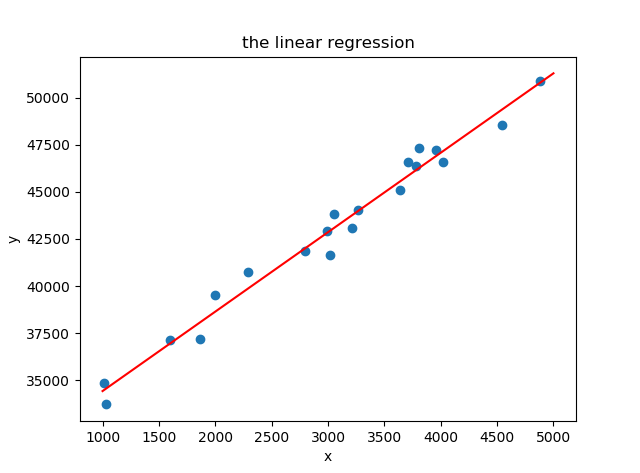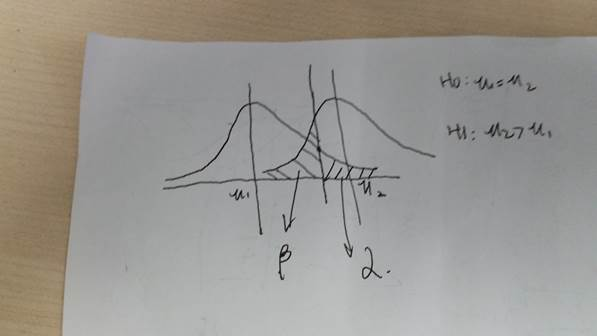• 数据可视化——R语言为ggplot图形添加P值和显著性水平准备安装和加载R包示例数据均值比较的方法用于添加P值的R函数compare_means()stat_compare_means()独立双样本间比较配对双样本间比较多样本的间比较多个...


数据可视化——R语言为ggplot图形添加P值和显著性水平
准备安装和加载R包示例数据
均值比较的方法用于添加P值的R函数compare_means()stat_compare_means()
独立双样本组间比较配对双样本组间比较多组样本的组间比较多个分组变量其他绘图方式

本文对一篇英文博客进行翻译，博客原文链接：Add P-values and Significance Levels to ggplots
概述：本文介绍如何轻松地为ggplot图形添加P值和显著性水平：
比较两组或多组的均值自动地将P值和显著性水平添加到ggplot图形中，如箱形图，点图，条形图和折线图等
使用工具： R语言中的ggplot2包和ggpubr包准备
安装和加载R包
本文使用ggpubr包，要求版本高于0.1.3。ggpubr是一个基于ggplot2的计算工具包。
直接输入以下命令从CARN中下载安装
install.packages("ggpubr")

也可以从GitHub中下载安装最新版本
if(!require(devtools)) install.packages("devtools")
devtools::install_github("kassambara/ggpubr")

加载ggpubr包
library(ggpubr)

ggpubr的官方文档可在以下位置获得：http://www.sthda.com/english/rpkgs/ggpubr
示例数据
示例数据集：ToothGrowth
data("ToothGrowth")

示例数据如下：
##    len supp dose
## 1  4.2   VC  0.5
## 2 11.5   VC  0.5
## 3  7.3   VC  0.5
## 4  5.8   VC  0.5
## 5  6.4   VC  0.5
## 6 10.0   VC  0.5

均值比较的方法
R中用于两组或多组间均值比较的标准统计方法在之前的文章也有描述：comparing means in R
均值比较的常见方法：
方法R实现函数描述T-testt.test()比较两组(参数检验)Wilcoxon testwilcox.test()比较两组(非参数检验)ANOVAaov()或anova()比较多组(参数检验)Kruskal-Walliskruskal.test()比较多组(非参数检验)
以下链接提供了各种方法的详细介绍：
单样本均值与已知均值的比较
单样本T检验（参数检验）  单样本Wilcoxon检验（非参数检验）   独立双样本均值比较
非配对双样本T检验（参数检验）非配对双样本Wilcoxon检验（非参数检验）  配对双样本均值比较
配对双样本T检验（参数检验）配对双样本Wilcoxon检验（非参数检验）  两组以上的均值比较
方差分析（ANOVA, 参数检验）
单因素方差分析双因素方差分析 Kruskal-Wallis检验（替代单因素方差分析的非参数检验）
用于添加P值的R函数
介绍两个ggpubr包中的函数
compare_means()：用于执行均值比较stat_compare_means()：用于在ggplot图形中自动添加P值和显著性水平
compare_means()
该函数用于执行均值比较。该函数与标准的R函数相比，灵活性更强。
简化形式如下：
compare_means(formula, data, method = "wilcox.test", paired = FALSE,
group.by = NULL, ref.group = NULL, ...)

formula：指定一个公式，公式形式为 x ~ group，其中，x 表示一个数值型变量，group 表示一个因子型变量，包含一个或多个水平。例如，一个示例公式为 formula = TP53 ~ cancer_group，表示在 cancer_group 对应的各水平间比较TP53的表达水平；也可以同时指定多个响应变量，如 formula = c(TP53, PTEN) ~ cancer_group。data：指定一个数据框(data.frame)，数据框需包含formula中的变量。method：指定统计检验的方法。默认为“wilcox.test”，即Wilcoxon检验（非参数检验）；也可指定其他统计方法：
“t.test”，即T检验（参数检验）。“t.test”和“wilcox.test”用于两组样本间的比较。当超过两组时，将会执行两两比较(pairwise comparison)。“anova”（参数检验）或 “kruskal.test”（非参数检验），用于执行多组间的单因素方差分析。 paired：指定一个逻辑变量，表示是否需要执行配对检验，仅适用于t.test 和wilcox.test。group.by：指定一个分组变量的字符名，用于在统计检验之前对数据进行分组。当存在group.by指定的变量时，均值比较将在不同水平的各个子集数据中执行。ref.group：指定一个组别的字符名，作为对照组(reference group)。如果指定，各个分组水平将与对照组水平进行比较。也可指定ref.group为“.all.”，表示每个分组水平将于所有分组水平（如base-mean）进行比较。
stat_compare_means()
该函数是对ggplot2的扩展，可将均值比较后的P值添加到ggplot图形中，如箱形图、点图、条形图和折线图等。
简化形式如下：
stat_compare_means(mapping = NULL, comparisons = NULL hide.ns = FALSE,
label = NULL,  label.x = NULL, label.y = NULL,  ...)

mapping：通过 aes() 设置绘图时的aesthetic  comparisons：指定一个列表(list)，每个列表元素需为长度等于2的向量。向量的内容可以为X轴的两个组别名（字符型），也可以是两个感兴趣组的组别索引（整数值），表示采用指定的两个组别进行比较。  hide.ns：逻辑变量，如果设为TRUE，显示显著性水平时将隐藏 ns 字样，即组间差异不显著时不显示 ns 字样。  label：指定一个字符串，表示标签类型。可为：“p.signif”（显示显著性水平），“p.format”（显示格式化的P值）。  label.x, label.y：指定一个数值，表示显示标签的绝对坐标位置。  …：传递给函数compare_means()的参数，如method、paired、ref.group。
独立双样本组间比较
执行统计检验
compare_means(len ~ supp, data = ToothGrowth)

示例结果如下：
## # A tibble: 1 x 8
##     .y. group1 group2      p  p.adj p.format p.signif   method
##
## 1   len     OJ     VC 0.0645 0.0645    0.064       ns Wilcoxon

method默认为“wilcox.test”（非参数检验），可指定method = “t.test”，表示T检验（参数检验）
返回值为具有以下列的数据框：
创建添加P值的箱形图
p <- ggboxplot(ToothGrowth, x = "supp", y = "len",
color = "supp", palette = "jco",
p + stat_compare_means()
# Change method
p + stat_compare_means(method = "t.test")注意：显示P值的标签位置可以通过如下参数来调整：label.x, label.y, hjust 和vjust。
显示P值的标签默认为 compare_means() 返回值中的 method 和 p 的组合。也可以通过 aes() 函数指定为其他显示形式。例如：
aes(label = ..p.format..) 或 aes(label = paste0(“p =”, ..p.format..))
表示只显示格式化的P值，而不显示method

aes(label = ..p.signif..)
表示仅显示显著性水平

aes(label = paste0(..method.., “\n”, “p =”, ..p.format..))
表示在method名和P值之间添加换行符(“\n”)

示例如下：
p + stat_compare_means( aes(label = ..p.signif..),
label.x = 1.5, label.y = 40)另外，也可以将参数label指定为字符向量：
p + stat_compare_means( label = "p.signif", label.x = 1.5, label.y = 40)

配对双样本组间比较
执行统计检验
compare_means(len ~ supp, data = ToothGrowth, paired = TRUE)

示例结果如下：
## # A tibble: 1 x 8
##     .y. group1 group2       p   p.adj p.format p.signif   method
##
## 1   len     OJ     VC 0.00431 0.00431   0.0043       ** Wilcoxon

使用函数 ggpaired() 可视化配对数据
ggpaired(ToothGrowth, x = "supp", y = "len",
color = "supp", line.color = "gray", line.size = 0.4,
palette = "jco")+
stat_compare_means(paired = TRUE)多组样本的组间比较
全局检验（所有组的均值比较）
# Global test
compare_means(len ~ dose,  data = ToothGrowth, method = "anova")

示例结果如下：
## # A tibble: 1 x 6
##     .y.        p    p.adj p.format p.signif method
##
## 1   len 9.53e-16 9.53e-16  9.5e-16     ****  Anova

添加全局检验的P值（所有组比较总的P值）
# Default method = "kruskal.test" for multiple groups
ggboxplot(ToothGrowth, x = "dose", y = "len",
color = "dose", palette = "jco")+
stat_compare_means()
# Change method to anova
ggboxplot(ToothGrowth, x = "dose", y = "len",
color = "dose", palette = "jco")+
stat_compare_means(method = "anova")两两比较(Pairwise comparisons)
如果分组变量包含两个以上的水平，两两比较的检验(pairwise test)将自动执行。默认方法为“wilcox.test”，也可设置为“t.test”。
# Perorm pairwise comparisons
compare_means(len ~ dose,  data = ToothGrowth)

示例结果如下：
## # A tibble: 3 x 8
##     .y. group1 group2        p    p.adj p.format p.signif   method
##
## 1   len    0.5      1 7.02e-06 1.40e-05  7.0e-06     **** Wilcoxon
## 2   len    0.5      2 8.41e-08 2.52e-07  8.4e-08     **** Wilcoxon
## 3   len      1      2 1.77e-04 1.77e-04  0.00018      *** Wilcoxon

# Visualize: Specify the comparisons you want
my_comparisons <- list( c("0.5", "1"), c("1", "2"), c("0.5", "2") )
ggboxplot(ToothGrowth, x = "dose", y = "len",
color = "dose", palette = "jco")+
stat_compare_means(comparisons = my_comparisons)+ # Add pairwise comparisons p-value
stat_compare_means(label.y = 50)     # Add global p-value如果需要指定标签显示的Y轴位置，可使用参数label.y
ggboxplot(ToothGrowth, x = "dose", y = "len",
color = "dose", palette = "jco")+
stat_compare_means(comparisons = my_comparisons, label.y = c(29, 35, 40))+
stat_compare_means(label.y = 45)注：ggsignif 包也可以很方便的为条形图添加组间比较的P值
相对于对照组的多重两两比较的检验(Multiple pairwise tests)
# Pairwise comparison against reference
compare_means(len ~ dose,  data = ToothGrowth, ref.group = "0.5",
method = "t.test")

示例结果如下：
## # A tibble: 2 x 8
##     .y. group1 group2        p    p.adj p.format p.signif method
##
## 1   len    0.5      1 6.70e-09 6.70e-09  6.7e-09     **** T-test
## 2   len    0.5      2 1.47e-16 2.94e-16  < 2e-16     **** T-test

# Visualize
ggboxplot(ToothGrowth, x = "dose", y = "len",
color = "dose", palette = "jco")+
stat_compare_means(method = "anova", label.y = 40)+      # Add global p-value
stat_compare_means(label = "p.signif", method = "t.test",
ref.group = "0.5")                    # Pairwise comparison against reference相对于所有组(base-mean)的多重两两比较的检验
# Comparison of each group against base-mean
compare_means(len ~ dose,  data = ToothGrowth, ref.group = ".all.",
method = "t.test")

示例结果如下：
## # A tibble: 3 x 8
##     .y. group1 group2        p    p.adj p.format p.signif method
##
## 1   len  .all.    0.5 1.24e-06 3.73e-06  1.2e-06     **** T-test
## 2   len  .all.      1 5.67e-01 5.67e-01     0.57       ns T-test
## 3   len  .all.      2 1.37e-05 2.74e-05  1.4e-05     **** T-test

# Visualize
ggboxplot(ToothGrowth, x = "dose", y = "len",
color = "dose", palette = "jco")+
stat_compare_means(method = "anova", label.y = 40)+      # Add global p-value
stat_compare_means(label = "p.signif", method = "t.test",
ref.group = ".all.")                  # Pairwise comparison against all下面使用Github中可用的骨髓瘤数据集展示一些典型情况，其中，与“.all.”的比较将很有用。
将依据患者molecular进行分组，绘制各个组别DEPDC1基因的表达水平。目的是比较各个组别之间是否存在差别，如果有差别，差别又在哪里。
要回答以上问题，可以在所有7个组之间进行两两比较(pairwise comparison)。 由于组别较多，将导致很多种组别组合，这将很难解释。
一个简单的解决办法是将7组中的每一组与“.all.”（如base-mean）比较。当检验结果显著时，可以得出结论：与所有组相比，xxx组的DEPDC1的表达水平显著降低或显著升高。
# Load myeloma data from GitHub
# Perform the test
compare_means(DEPDC1 ~ molecular_group,  data = myeloma,
ref.group = ".all.", method = "t.test")

示例结果如下：
## # A tibble: 7 x 8
##      .y. group1           group2        p   p.adj p.format p.signif method
##
## 1 DEPDC1  .all.       Cyclin D-1 0.149690 0.44907  0.14969       ns T-test
## 2 DEPDC1  .all.       Cyclin D-2 0.523143 1.00000  0.52314       ns T-test
## 3 DEPDC1  .all.     Hyperdiploid 0.000282 0.00169  0.00028      *** T-test
## 4 DEPDC1  .all. Low bone disease 0.005084 0.02542  0.00508       ** T-test
## 5 DEPDC1  .all.              MAF 0.086107 0.34443  0.08611       ns T-test
## 6 DEPDC1  .all.            MMSET 0.576291 1.00000  0.57629       ns T-test
## # ... with 1 more rows

注意：上面的R代码中myeloma数据的下载地址存在错误，可从以下链接找到myeloma数据：https://github.com/kassambara/data/blob/master/myeloma.txt 然后自己将全部数据复制并保存为myeloma.txt，从本地读取myeloma.txt。
# Visualize the expression profile
ggboxplot(myeloma, x = "molecular_group", y = "DEPDC1", color = "molecular_group",
add = "jitter", legend = "none") +
rotate_x_text(angle = 45)+
geom_hline(yintercept = mean(myeloma$DEPDC1), linetype = 2)+ # Add horizontal line at base mean stat_compare_means(method = "anova", label.y = 1600)+ # Add global annova p-value stat_compare_means(label = "p.signif", method = "t.test", ref.group = ".all.") # Pairwise comparison against all根据上图的结果，可以得出proliferation组的DEPDC1表达水平显著升高；Hyperdiploid组和Low bone disease组中DEPDC1表达水平显著降低。 注意：想要隐藏 ns 标志，可以设置参数 hide.ns = TRUE # Visualize the expression profile ggboxplot(myeloma, x = "molecular_group", y = "DEPDC1", color = "molecular_group", add = "jitter", legend = "none") + rotate_x_text(angle = 45)+ geom_hline(yintercept = mean(myeloma$DEPDC1), linetype = 2)+ # Add horizontal line at base mean
stat_compare_means(method = "anova", label.y = 1600)+        # Add global annova p-value
stat_compare_means(label = "p.signif", method = "t.test",
ref.group = ".all.", hide.ns = TRUE)      # Pairwise comparison against all多个分组变量
使用另一个变量进行分组后再执行独立双样本比较
执行统计检验：
compare_means(len ~ supp, data = ToothGrowth,
group.by = "dose")

示例结果如下：
## # A tibble: 3 x 9
##    dose   .y. group1 group2       p  p.adj p.format p.signif   method
##
## 1   0.5   len     OJ     VC 0.02319 0.0464    0.023        * Wilcoxon
## 2   1.0   len     OJ     VC 0.00403 0.0121    0.004       ** Wilcoxon
## 3   2.0   len     OJ     VC 1.00000 1.0000    1.000       ns Wilcoxon

在上面的示例中，对于分类变量dose的每一个水平，分类变量supp又将数据分为两个水平：OJ和VC，然后在这两个水平上对数值变量len进行均值比较。
可视化：创建一个按组划分的多面板框图(此处为“dose”)
# Box plot facetted by "dose"
p <- ggboxplot(ToothGrowth, x = "supp", y = "len",
color = "supp", palette = "jco",
facet.by = "dose", short.panel.labs = FALSE)
# Use only p.format as label. Remove method name.
p + stat_compare_means(label = "p.format")# Or use significance symbol as label
p + stat_compare_means(label =  "p.signif", label.x = 1.5)注意：想要隐藏 ns 标志，可以设置参数hide.ns = TRUE
可视化：创建一个包含所有箱形图的单一面板。X表示dose，Y表示len，颜色表示supp
p <- ggboxplot(ToothGrowth, x = "dose", y = "len",
color = "supp", palette = "jco",
p + stat_compare_means(aes(group = supp))# Show only p-value
p + stat_compare_means(aes(group = supp), label = "p.format")# Use significance symbol as label
p + stat_compare_means(aes(group = supp), label = "p.signif")使用另一个变量进行分组后再执行配对双样本比较
执行统计检验：
compare_means(len ~ supp, data = ToothGrowth,
group.by = "dose", paired = TRUE)

实例结果如下：
## # A tibble: 3 x 9
##    dose   .y. group1 group2      p  p.adj p.format p.signif   method
##
## 1   0.5   len     OJ     VC 0.0330 0.0659    0.033        * Wilcoxon
## 2   1.0   len     OJ     VC 0.0191 0.0572    0.019        * Wilcoxon
## 3   2.0   len     OJ     VC 1.0000 1.0000    1.000       ns Wilcoxon

可视化：创建一个按组划分的多面板框图(此处为“dose”)
# Box plot facetted by "dose"
p <- ggpaired(ToothGrowth, x = "supp", y = "len",
color = "supp", palette = "jco",
line.color = "gray", line.size = 0.4,
facet.by = "dose", short.panel.labs = FALSE)
# Use only p.format as label. Remove method name.
p + stat_compare_means(label = "p.format", paired = TRUE)其他绘图方式
条形图和折线图（一个分组变量）
# Bar plot of mean +/-se
ggbarplot(ToothGrowth, x = "dose", y = "len", add = "mean_se")+
stat_compare_means() +                                         # Global p-value
stat_compare_means(ref.group = "0.5", label = "p.signif",
label.y = c(22, 29))                   # compare to ref.group
# Line plot of mean +/-se
ggline(ToothGrowth, x = "dose", y = "len", add = "mean_se")+
stat_compare_means() +                                         # Global p-value
stat_compare_means(ref.group = "0.5", label = "p.signif",
label.y = c(22, 29))条形图和折线图（两个分组变量）
ggbarplot(ToothGrowth, x = "dose", y = "len", add = "mean_se",
color = "supp", palette = "jco",
position = position_dodge(0.8))+
stat_compare_means(aes(group = supp), label = "p.signif", label.y = 29)
ggline(ToothGrowth, x = "dose", y = "len", add = "mean_se",
color = "supp", palette = "jco")+
stat_compare_means(aes(group = supp), label = "p.signif",
label.y = c(16, 25, 29))注意：经过实际测试，笔者发现R语言中的统计方法计算结果的P值与SPSS中的P值存在差异。如，常规的方差分析(ANOVA) + 事后两两组间比较（如Bonferroni校正）使用上述R函数就很难得出与SPSS中一致的结果。如果需要使用SPSS的统计P值，建议对生成的图形进行后期修改。
References
原文链接：Add P-values and Significance Levels to ggplots
展开全文R语言 ggplot2
• 现有两组数据长度L和弹性E，分析其相关性，想找到长度L为多少时，弹性E最大，在graphpad中得到结果是线性相关，却无法给出想要的L最优。用什么统计方法能解决此问题呢？ Number of XY Pairs 5332 Pearson r -0....相关性
• 本文主要讨论Python实现一元回归的线性拟合、最小二乘法估计回归参数和显著性检验（t检验和p值）. 一元线性回归模型是描述两个变量之间相关关系的最简单的回归模型. 通常人们对所要研究的问题首先要收集与它有关的n...

本文主要讨论Python实现一元回归的线性拟合、最小二乘法估计回归参数和显著性检验（t检验和p值）.

一元线性回归模型是描述两个变量之间相关关系的最简单的回归模型. 通常人们对所要研究的问题首先要收集与它有关的n组样本数据（x_i,y_i），i=1,2,3...，为了直观的发现样本数据的分布规律，把（x_i,y_i）看成是平面直角坐标系中的点. 描述两个变量之间的线性关系的数学结构通常为：
y=β_0+β_1*x+e
其中，β_0+β_1*x是由于x的变化引起y线性变化的部分，e是随机因素引起的部分.

from scipy import stats
import pandas as pd
import numpy as np
import matplotlib.pyplot as plt

#读取数据并查看数据的前五行,sheetname=n，其中n表示excel中的工作表的索引（即第n+1个工作表）

#计算x,y的平均值
x=df['x']
y=df['y']
#画出x与y的散点图
plt.scatter(x,y)
plt.xlabel('x')
plt.ylabel('y')
plt.title('the linear regression')
#线性回归拟合
x_m=np.mean(x)
y_m=np.mean(y)
x1=(x-x_m)
y1=y-y_m
x2=sum((x-x_m)**2)
xy=sum(x1*y1)
#回归参数的最小二乘估计
beta1=xy/x2
beta0=y_m-beta1*x_m
#输出线性回归方程
print('y=',beta0,'+',beta1,'*x')
#画出回归方程的函数图
a=np.linspace(1000,5000,1000)#b表示在(1000,5000)上生成1000个a值
b=[beta0+beta1*i for i in a]
plt.plot(a,b,'r')
#方差
sigma2=sum((y-beta0-beta1*x)**2)/(18)
#标准差
sigma=np.sqrt(sigma2)
#求t值
t=beta1*np.sqrt(x2)/sigma
print('t=',t)
#已知临界值求p值
p=stats.t.sf(t,18)
print('p=',p)

#输出检验结果
if p<0.05:
print ('the linear regression between x and y is significant')
else:
print('the linear regression between x and y is not significant')

plt.show()
执行结果：展开全文Python 线性回归 最小二乘法 t检验
• 最近一直在学算法，c语言的指针在算法中用的不多，所以就没怎么学，直到后来帮我同学做课程设计的时候，才学了段时间，为了防止过段时间忘了指针，所以就把课程设计保存下来，以后忘了还可以看看这个回忆一下指针...
最近一直在学算法，c语言的指针在算法中用的不多，所以就没怎么学，直到后来帮我同学做课程设计的时候，才学了一段时间，为了防止过段时间忘了指针，所以就把课程设计保存下来，以后忘了还可以看看这个回忆一下指针。
#include <stdio.h>

int main()
{
int i,sum,a,b,flag;
double q;
int *max1,*min1;
sum=0;flag=0;
for(i=0;i<10;i++)     //输入十个数
{
if(flag==1)
{
int *p=NULL,temp;//初始化指针
scanf("%d",&a[i]);
sum+=a[i];
p=&a[i];
if(*p>max1)//求最大值
{
max1=*p;
}
if(*p<min1)//求最小值
{
min1=*p;
}
}
if(flag==0)
{
scanf("%d%d",&a,&b);//求出第一和第二个值的最大值和最小值作为初始值
sum+=a;
sum+=b;
max1=a>b?a:b;
min1=a>b?b:a;
flag=1;
}
}
q=(double)sum/10;//变格式（并求出平均值）
printf("%d %d %.2lf",min1,max1,q);//输出（最小值，最大值，平均值）
}

展开全文• ## 理解P值

千次阅读 2016-06-03 17:10:30
P值的范围0到1，是个概率，用来度量否定原假设的证据，概率越低，否定原假设的证据越充分。可以将P值与显著性水平α（significance level）相比较，P值小于等于α，则否定H0；否则，无法否定H0. α反映当H0为真时...
      P值是 一种概率，一种在原假设为真的前提下出现观察样本以及更极端情况的概率。

P值的计算：

一般地，用X 表示检验的统计量，当H0为真时，可由样本数据计算出该统计量的值C，根据
检验统计量X的具体分布，可求出P值。具体地说：

左侧检验的P值为检验统计量X 小于样本统计值C 的概率，即：P = P{ X < C}

右侧检验的P值为检验统计量X 大于样本统计值C 的概率：P = P{ X > C}

双侧检验的P值为检验统计量X 落在样本统计值C 为端点的尾部区域内的
概率的2 倍：P = 2P{ X > C} (当C位于分布曲线的右端时) 或P = 2P{ X< C} (当C 位于分布曲线的左端时) 。若X 服从
正态分布和t分布，其分布曲线是关于纵轴对称的，故其P 值可表示为P = P{| X| > C} 。

P值的范围0到1，是一个概率，用来度量否定原假设的证据，概率越低，否定原假设的证据越充分。可以将P值与显著性水平α（significance level）相比较，P值小于等于α，则否定H0；否则，无法否定H0. α反映当H0为真时拒绝H0的概率，也就是犯第一类错误的概率。
显著性水平（alpha）是当零假设为真时拒绝它的概率。
当零假设为假时接受它的概率为(beta),拒绝它的概率为1-beta,检验能力power of test 的值为 1- beta
一类错误和二类错误是负相关的，有时我们需要考虑哪一类错误后果更严重，从而选择合适的显著性水平和检验能力。例如为了检验新药是否和原药更效果一样，零假设为效果相等，备则假设为效果不同，犯第一类错误表明该药其实和原药效果相等，但误以为更有效（假阳性错误），犯第二类错误表明该药本来效果不同，但因为不能拒绝（接受）了零假设，而认为效果相同。犯第一类错误，后果就是病人吃了和原来效果一样的药，没有生命危险；犯第二类错误，后果就是把未知效果的药给病人吃了，后果很严重。P值表示当H0假设为真时，你的样本数据出现的概率。注意：常见错误是把P值理解为0假设为真的概率。假设P值为0.03,就是说你有3%的概率观察到当前样本数据与H0假设值的差异或者更大的差异。注意它不是指你犯第一类错误的概率为3%。当P值为0.05是，错误拒真（假阳性falsepositive）的概率至少23%，一般接近50%；当P值为0.01时，错误拒真的概率至少7%，一般接近15%。所有当P值是0.05时，样本数据中观察到的差异可能在总体中并不存在。
如果一次实验中，P值为0.05，不太可能降低falsepositive rate.但是独立重复实验却是可以的，如果两次独立并不是刻意挑出的实验P值都是0.05，可以得出P*P=0.025的新P值
如果想从检测到一个效果移步到评估它的大小和精度，使用置信区间。
备则假设也很重要：当P值为0.05时，如果备则假设出现概率很高，则相关的错误率至少12%；而如果备则假设几乎没出现过，则相关的错误率至少76%。

P的意义不表示两组差别的大小，P反映两组差别有无统计学意义，并不表示差别大小。因此，与对照组相比，C药取得P<0.05，D药取得P <0.01并不表示D的药效比C强。P>0.05时，差异无显著意义，根据统计学原理可知，不能否认无效假设，但并不认为无效假设肯定成立。在药效统计分析中，更不表示两药等效。哪种将“两组差别无显著意义”与“两组基本等效”相同的做法是缺乏统计学依据的。


展开全文• 在很多模型及假设检验中都需要满足个假设条件：数据需服从正态分布。这篇文章主要讲讲如何判断数据是否符合正态分布。主要分为两种方法：描述统计方法和统计检验方法。 描述统计方法 描述统计就是用描述的数字或...python 统计学
• p值 统计学意义P values are one of the most widely used concepts in statistical analysis. They are used by researchers, analysts and statisticians to draw insights from data and make informed decisions...python 机器学习 java 人工智能 大数据
• 测试一组数据是否来自某一泊松分布data (n = 100, 20) mean (data) poisson.test(sum(data), length(data), mean)# Exact Poisson test # # data: sum(data) time base: length(data) # number of events = 2000,
• 或许当你看到行代码的...）就像进行数据处理的时候，有时会遇到极值（最大、最小值）、平均、中位数和四分位数（25%、 75%）的情况。 这篇博客就是你的福音，让你绝对0基础使用python 进行数据分析。 ...
• # -*-coding: utf-8 -*- import pandas as pd #读取csv文件 df=pd.read_csv('A_2+20+DoW+VC.csv') ...#把ave_time这列的缺失进进行填充，填充的方法是按这列的平均进行填充 df2=df.fillna(aveTime) #pandas
• 打开Minitab之后 点击Stat>Basic Statistics> Normality Test  分析之后若 P value(P值)>0.05，说明此组数据服从正态分布测试 正态分布
• 用SAS、SPSS等专业统计软件进行假设检验，在假设检验中常见到P值( P-Value，Probability，Pr)，P值是进行检验决策的另个依据。P值即概率，反映某事件发生的可能性大小。统计学根据显著性检验方法所得到的P 值，...
• 正态分布： 若随机变量x服从有个数学期望为μ,方差为σ2的正态分布，记为N(μ,σ) 其中期望决定密度函数的位置，标准差决定分布的幅度，当υ=0，σ=0 时的...#构造一组随机数据 s = pd.DataFrame(np.random.randn.
• ## 【数理统计】神奇的P值

千次阅读 多人点赞 2019-06-29 13:46:21
工作中经常会通过AB Test帮助做产品决策，简单说就是为产品制作两个（A/B）或多个（A/B/C/...）版本，在同一时间维度，分别让不同的用户群随机的访问这些版本，收集各群组的用户的数据，最后分析评估出最好版本...
• 在工作中遇到了一个需求，需要先GroupBy再获取每一组的最后一条 首先这里有一个基础知识： 如果groupby之后select之内没有聚合函数操作，则输出每一组的第一条 所以说为了获取每一组的最后一条，只需要用orderby...
• 用多项式函数（10.2）作为插值函数时，希望通过解方程（10.3）而得到待定系数 function y=lagrange(x0,y0,x); n=length(x0);m=length(x); for i=1:m z=x(i); s=0.0; for k=1:n p=1.0; for j=1:n if j...
• 思考：输入到NN模型中的特征要做归一化处理...言归正传，airbnb根据不同特征做不一样的归一化，因为他们对数据进行了观察，发现了部分长尾数据，因此做了log的归一化处理，这点很惊喜。在我刚工作的时候，也有人...分布 分布检验
• 数据挖掘：数据预处理——缺失处理 、什么是缺失 缺失是指粗糙数据中由于缺少信息而造成的数据的聚类、分组、删失或截断。它指的是现有数据集中某个或某些属性的是不完全的。 而在数据处理的过程中，缺失...机器学习 python
• 数据的准备往往占到...将存在遗漏信息属性数据删除，得到个完整的数据组。 优点：简单易行，在数据含有多个属性缺失、被删除的含缺失数据与信息表中的数据量相比非常小的情况下是非常有效的 缺点：...机器学习
• ANOVA(analysis of variance)，方差分析，曾经以为它是个多么复杂的简写。。。   、理解F分布 要理解F分布，就要先理解卡方分布，要理解卡方分布，就要先理解正态分布。 1.正态分布的概率密度函数的表达式...ANOVA
• 通常，我们倾向于在构建模型时忽略异常，这不是个明智的做法， 异常使数据偏移并降低准确性，在此让我们进一步了解异常处理。 什么样的是异常？ 异常是分析师和数据科学家常用的术语，因为它需要密切...
• ## numpy求平均值

千次阅读 2020-08-28 14:32:44
1.对数组求平均 import numpy as np a = np.array([[1, 2], [3, 4]]) print(a) print(type(a)) print(np.mean(a)) print(np.mean(a, axis=0)) # axis=0，计算每列的均值 print(np.mean(a, axis=1)) # 计算每...python numpy
• 上篇讲了P值与Z得分的一些基本概念，大家其实也都知道，P值和Z得分其实是有一定的相应关系的，Z得分有正负两种结果，而P值有显著和不显著两种可能。 如果按照我们一般的思维，P值和Z得分...上篇文章说过了，P值是用空间统计 空间自相关 算法 聚类
• 数据预处理是明确分析目标与思路之后进行数据分析的第步，也是整个项目中最基础、花费时间较长的工作。除了互联网埋点的数据或企业内部的业务数据之外，往往我们拿到的，比如说网上采集的数据并不是那样规整，这类...python 统计学
• 数据很多，比如有几千万个，要求均值，全部数据都是整数，范围0 ~ 255如果简单的累加起来，使用long类型，加完之后再除以数据总个数，这样均值，求和时会溢出啊。有什么办法么？避免溢出的同时，还要保证数据的...算法 优化 测试 java
• ## R语言 处理缺失值数据

万次阅读 多人点赞 2016-05-18 11:34:55r语言
• P1849表达式求值 Accepted 标签：NOIP普及2013 描述 给定个只包含加法和乘法的算术表达式,请你编程计算表达式的。 格式 输入格式 输入仅有行,为需要你计算的表达式,表达式中只包含...
• 1.原始数据是乱序的 2.原始数据是有序的 1. 1 package test; 2 3 import java.io.File; 4 5 public class Test { 6 /** 7 * 假设原始数据是乱序 8 */ 9 static int[] src = new int[] { ....
• 异常分析是检验数据是否有录入错误数据和不合常理的数据。不加剔除的把异常代入数据分析过程中，会对结果产生不良影响，而对异常的分析其原因，常常成为为发现问题的而改进决策的契机。 异常是指样本中的...python 大数据 箱型图...